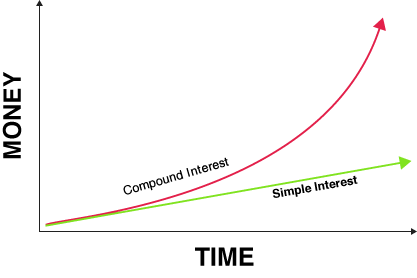# Future Value of Money

The money has time value. A rupee today is more valuable than a rupee one year hence. The inflation reduces the purchasing power of money. In this article we will understand about the future value of money.

The future value of money depends upon the type of interest accrued on investment. There are two method of calculating interest:

1. Simple Interest
2. Compound Interest### Future value of money by Simple Interest

In simple interest, the interest earned on investment is accrued uniformly during the period of investment.

Suppose you invest Rs 1,000 for five year in saving account that pays 10% simple interest per year. Then the value of investment after five year will be as follows:

 Year Principle Interest for year Ending Balance 1 1000 100 1100 2 1000 100 1200 3 1000 100 1300 4 1000 100 1400 5 1000 100 1500

Here you see that Principle remains same throughout the period of investment.

The general formula for calculating the future value of money invested today at simple interest is:

Future value = Present Value [1 + Number of year x Interest Rate]

Also written in short form as:

FVn = PV (1 + nr)

Where:

PV = Present value
FVn = Future value of money n years hence
r = Interest Rate
n = number of years

### Future value of money by Compound Interest

If the money is invested at compound interest then the interest is reinvested to earn further interest in future periods.

Suppose you invest Rs 1,000 for five year in saving account that pays 10% compound interest per year. Then the value of investment after five year will be as follows:

 Year Principle Interest for year Ending Balance 1 1000 100 1100 2 1100 110 1210 3 1210 121 1331 4 1331 133.10 1464.10 5 1464.10 146.41 1610.51

The general formula for calculating the future value of money invested today at simple interest is:

Future value = Present Value [1 + Interest Rate]Number of years

Also written in short form as:

FVn = PV (1 + r)n

Where

PV = Present value
FVn = Future value of money n years hence
r = Interest Rate
n = number of years

### Power of Compounding

Let’s now compare the difference between simple interest and compound interest through the table given below:

 Year Ending Balance at Simple interest Ending Balance at Compund interest 1 1100 1100 2 1200 1210 3 1300 1331 4 1400 1464.10 5 1500 1610.51

You must have understood the power of compounding. Einstein has once described compound interest as “the eighth wonder of the world”.

Under simple interest the growth is linear whereas under compound interest the growth is exponential.### Rule of 72

People commonly ask the question: How long would it take to double the amount at a given rate of interest? The rule of 72 answers this question. According to this rule, the doubling period is obtained by dividing 72 by the interest rate. Suppose if interest rate is 9 percent, then the double period is about 72/9 = 8 years. Thus it takes 8 years to double the amount at 9 percent rate of interest.

We hope you liked this article on Future Value of Money. Here are some useful articles for you to read next: